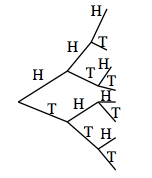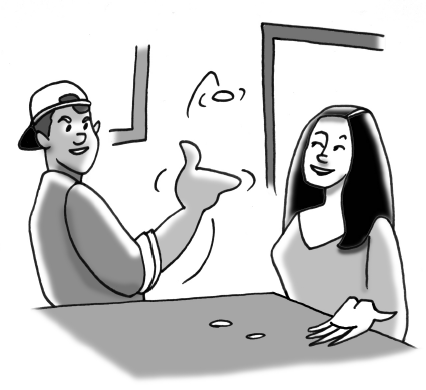### Home > CCG > Chapter 4 > Lesson 4.2.2 > Problem4-69

4-69.

Eddie is arguing with Tana about the probability of flipping three coins. They decided to flip a penny, nickel, and a dime.

1. Which would be better for determining the sample space, a tree diagram or an area model? Justify your answer.

How many dimensions are needed?

Tree diagram, but why?

2. Make a sample space that shows all the possible outcomes. How many outcomes are there?3. Find the probability of each of the following events occurring. Be sure to show your thinking clearly:

1. One head and two tails

1. At least one tail

1. Exactly two tails

Use the diagram from part (b) to answer (i) − (iv).

4. Which is more likely, flipping at least $2$ heads or at least $2$ tails? Explain.

Refer to the diagram.

5. How would the probabilities change if Tana found out that Eddie was using weighted coins (coins that were not fair) so that the probability of getting heads for each coin was $\frac { 4 } { 5 }$ instead of $\frac { 1 } { 2 }$? Would this change the sample space? Recalculate the probabilities in part (c) based on the new information.

The sample space remains the same but the probabilities would change.Use the eTool below to test probabilities.
Click on the link at right for the full version of the eTool: CCG 4-69 HW eTool